Скачать презентацию Foreign Exchange Markets Dr Bryan Mills Based on

02e454bc3b3ba1e292e467abb5397f90.ppt

• Количество слайдов: 31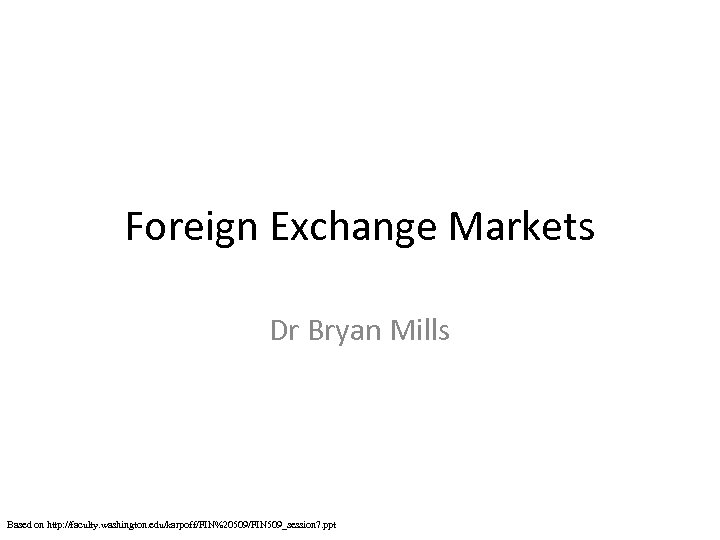Foreign Exchange Markets Dr Bryan Mills Based on http: //faculty. washington. edu/karpoff/FIN%20509/FIN 509_session 7. ppt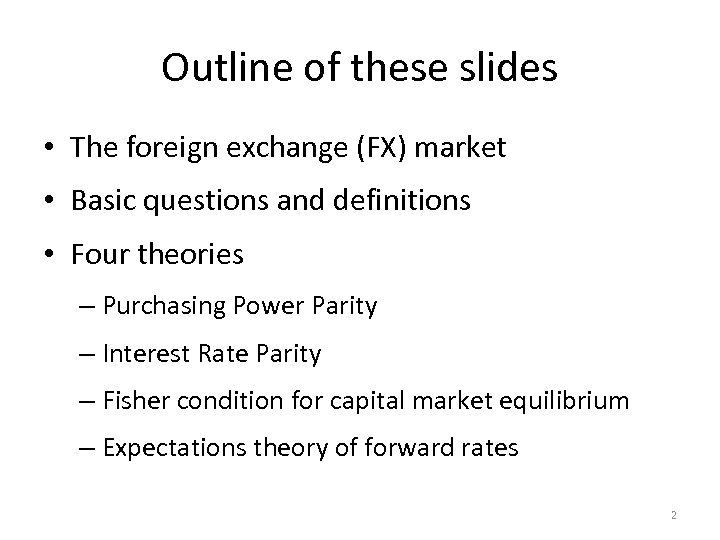Outline of these slides • The foreign exchange (FX) market • Basic questions and definitions • Four theories – Purchasing Power Parity – Interest Rate Parity – Fisher condition for capital market equilibrium – Expectations theory of forward rates 2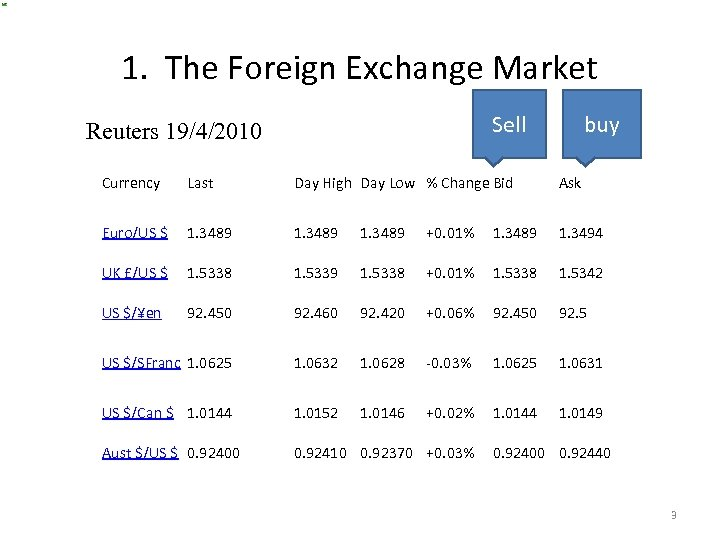1. The Foreign Exchange Market Sell Reuters 19/4/2010 buy Currency Last Day High Day Low % Change Bid Ask Euro/US \$ 1. 3489 +0. 01% 1. 3489 1. 3494 UK £/US \$ 1. 5338 1. 5339 1. 5338 +0. 01% 1. 5338 1. 5342 US \$/¥en 92. 450 92. 460 92. 420 +0. 06% 92. 450 92. 5 US \$/SFranc 1. 0625 1. 0632 1. 0628 -0. 03% 1. 0625 1. 0631 US \$/Can \$ 1. 0144 1. 0152 1. 0146 +0. 02% 1. 0144 1. 0149 Aust \$/US \$ 0. 92400 0. 92410 0. 92370 +0. 03% 0. 92400 0. 92440 3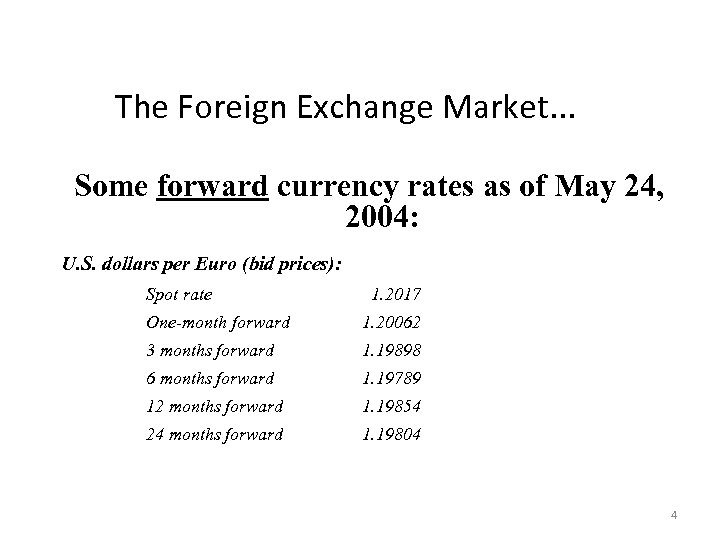The Foreign Exchange Market. . . Some forward currency rates as of May 24, 2004: U. S. dollars per Euro (bid prices): Spot rate 1. 2017 One-month forward 1. 20062 3 months forward 1. 19898 6 months forward 1. 19789 12 months forward 1. 19854 24 months forward 1. 19804 4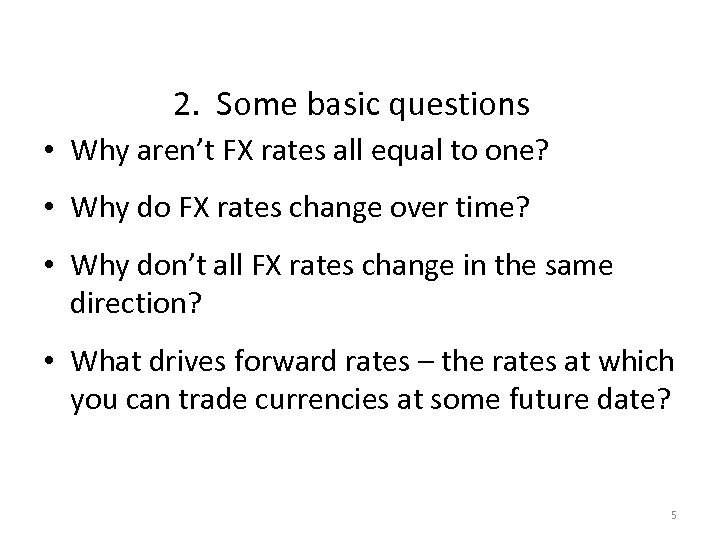2. Some basic questions • Why aren’t FX rates all equal to one? • Why do FX rates change over time? • Why don’t all FX rates change in the same direction? • What drives forward rates – the rates at which you can trade currencies at some future date? 5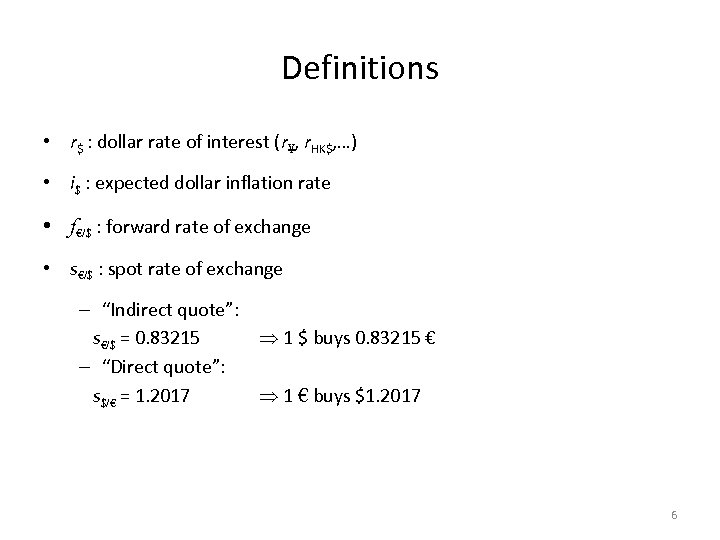Definitions • r\$ : dollar rate of interest (r¥, r. HK\$, …) • i\$ : expected dollar inflation rate • f€/\$ : forward rate of exchange • s€/\$ : spot rate of exchange – “Indirect quote”: s€/\$ = 0. 83215 1 \$ buys 0. 83215 € – “Direct quote”: s\$/€ = 1. 2017 1 € buys \$1. 2017 6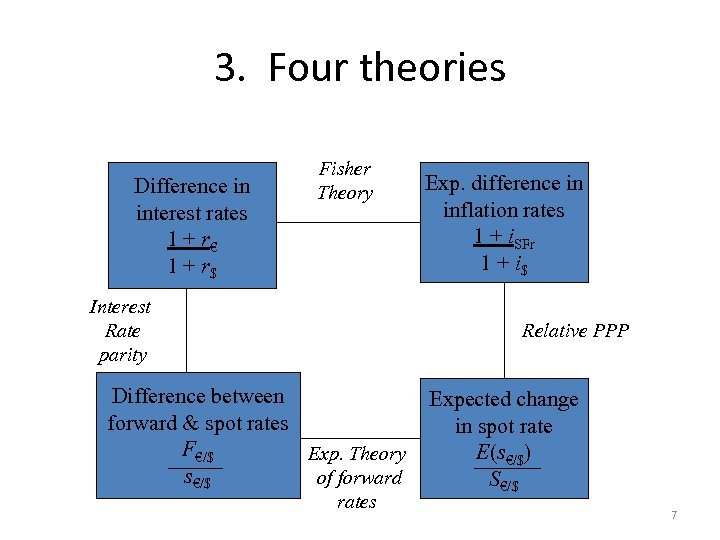3. Four theories. Difference in interest rates 1 + r€ 1 + r\$ Fisher Theory Interest Rate parity Exp. difference in inflation rates 1 + i. SFr 1 + i\$ Relative PPP Difference between forward & spot rates F€/\$ Exp. Theory s€/\$ of forward rates Expected change in spot rate E(s€/\$) S€/\$ 7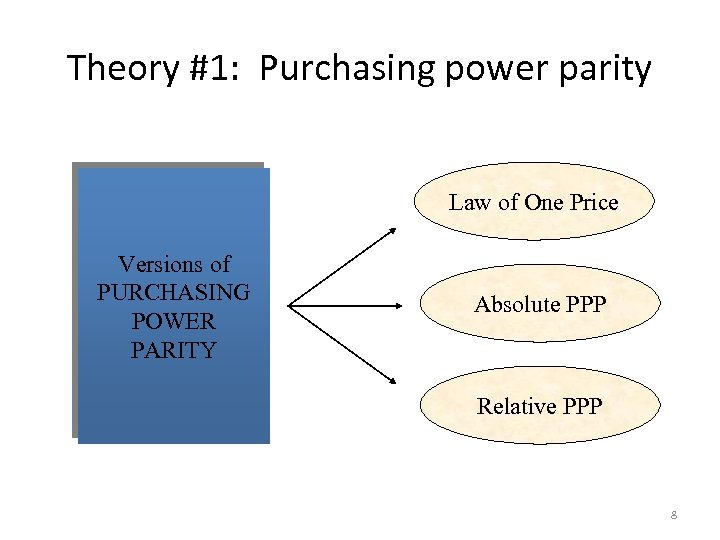Theory #1: Purchasing power parity Law of One Price Versions of PURCHASING POWER PARITY Absolute PPP Relative PPP 8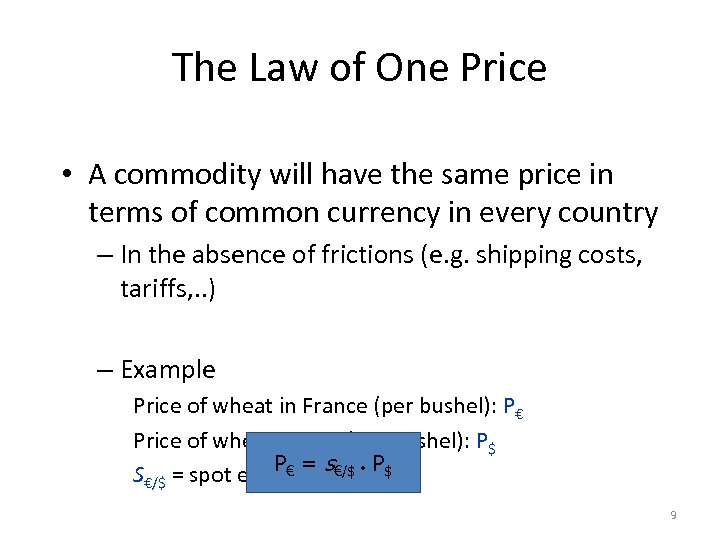The Law of One Price • A commodity will have the same price in terms of common currency in every country – In the absence of frictions (e. g. shipping costs, tariffs, . . ) – Example Price of wheat in France (per bushel): P€ Price of wheat in U. S. (per bushel): P\$ P€ = s S€/\$ = spot exchange €/\$ P\$ rate 9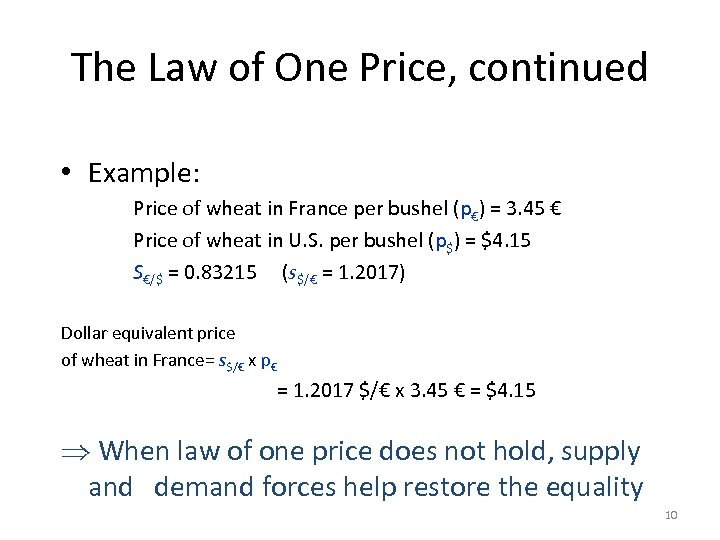The Law of One Price, continued • Example: Price of wheat in France per bushel (p€) = 3. 45 € Price of wheat in U. S. per bushel (p\$) = \$4. 15 S€/\$ = 0. 83215 (s\$/€ = 1. 2017) Dollar equivalent price of wheat in France= s\$/€ x p€ = 1. 2017 \$/€ x 3. 45 € = \$4. 15 When law of one price does not hold, supply and demand forces help restore the equality 10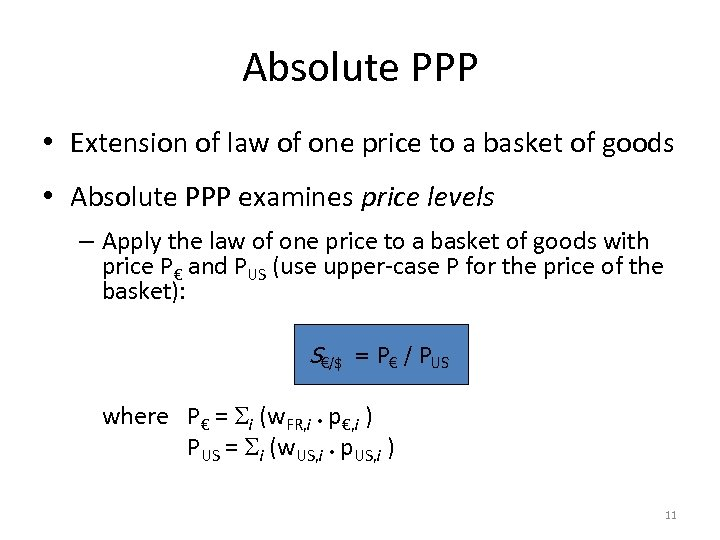Absolute PPP • Extension of law of one price to a basket of goods • Absolute PPP examines price levels – Apply the law of one price to a basket of goods with price P€ and PUS (use upper-case P for the price of the basket): S€/\$ = P€ / PUS where P€ = i (w. FR, i p€, i ) PUS = i (w. US, i p. US, i ) 11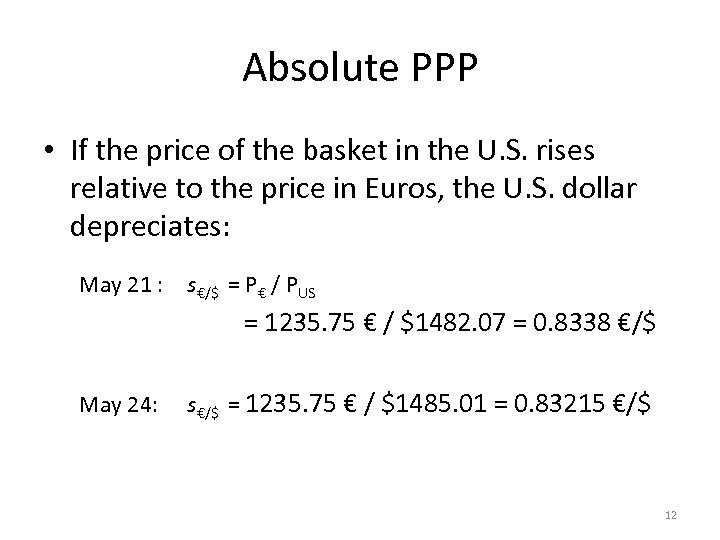Absolute PPP • If the price of the basket in the U. S. rises relative to the price in Euros, the U. S. dollar depreciates: May 21 : s€/\$ = P€ / PUS = 1235. 75 € / \$1482. 07 = 0. 8338 €/\$ May 24: s€/\$ = 1235. 75 € / \$1485. 01 = 0. 83215 €/\$ 12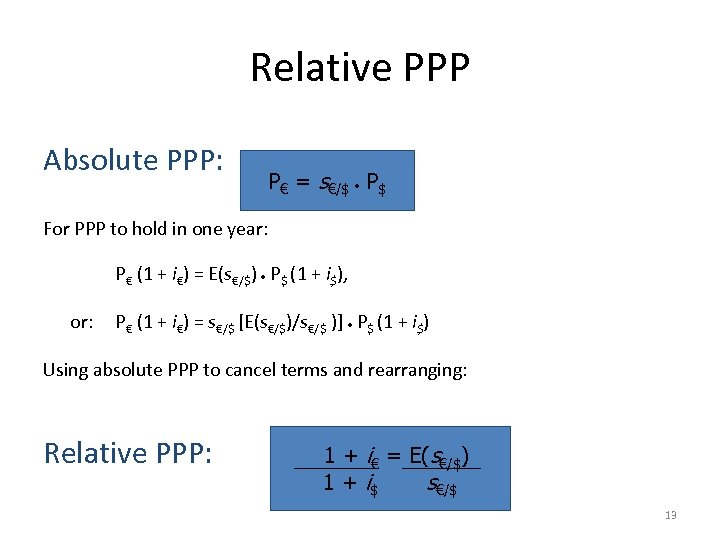Relative PPP Absolute PPP: P€ = s€/\$ P\$ For PPP to hold in one year: P€ (1 + i€) = E(s€/\$) P\$ (1 + i\$), or: P€ (1 + i€) = s€/\$ [E(s€/\$)/s€/\$ )] P\$ (1 + i\$) Using absolute PPP to cancel terms and rearranging: Relative PPP: 1 + i€ = E(s€/\$) 1 + i\$ s€/\$ 13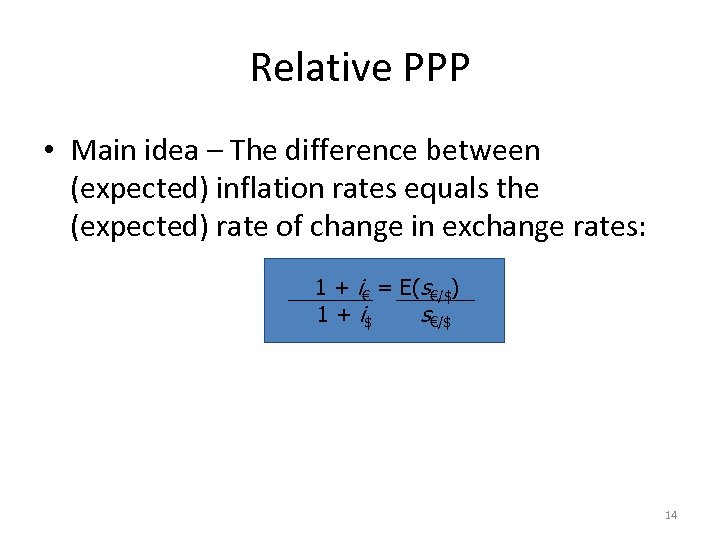Relative PPP • Main idea – The difference between (expected) inflation rates equals the (expected) rate of change in exchange rates: 1 + i€ = E(s€/\$) 1 + i\$ s€/\$ 14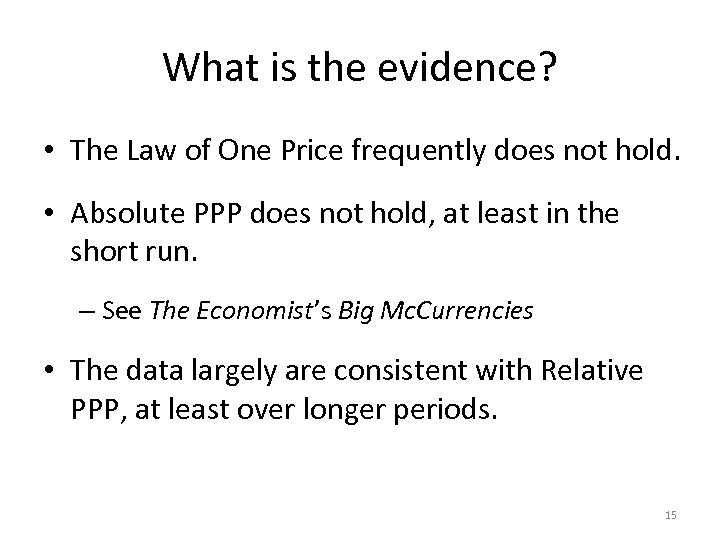What is the evidence? • The Law of One Price frequently does not hold. • Absolute PPP does not hold, at least in the short run. – See The Economist’s Big Mc. Currencies • The data largely are consistent with Relative PPP, at least over longer periods. 15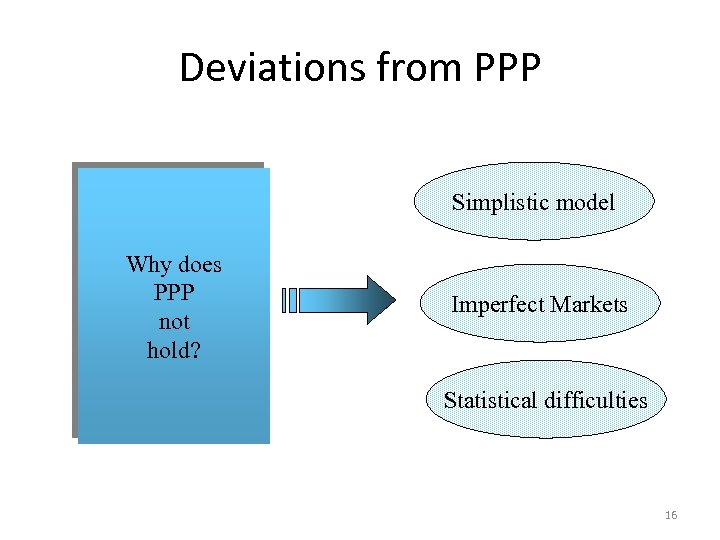Deviations from PPP Simplistic model Why does PPP not hold? Imperfect Markets Statistical difficulties 16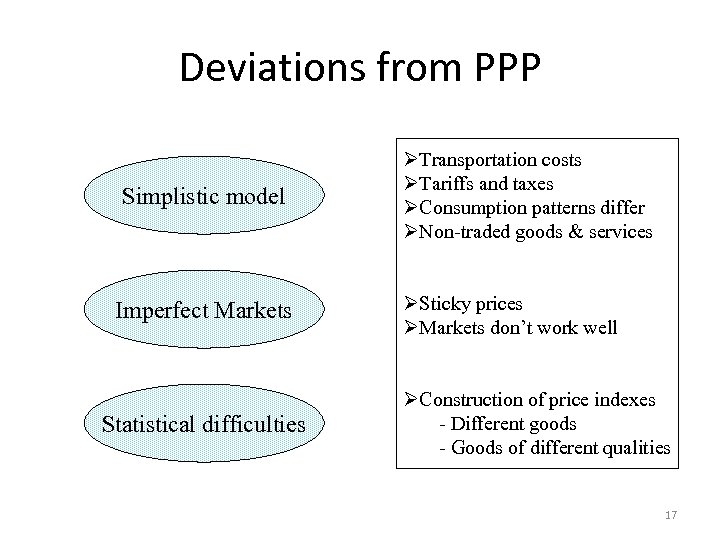Deviations from PPP Simplistic model Imperfect Markets Statistical difficulties ØTransportation costs ØTariffs and taxes ØConsumption patterns differ ØNon-traded goods & services ØSticky prices ØMarkets don’t work well ØConstruction of price indexes - Different goods - Goods of different qualities 17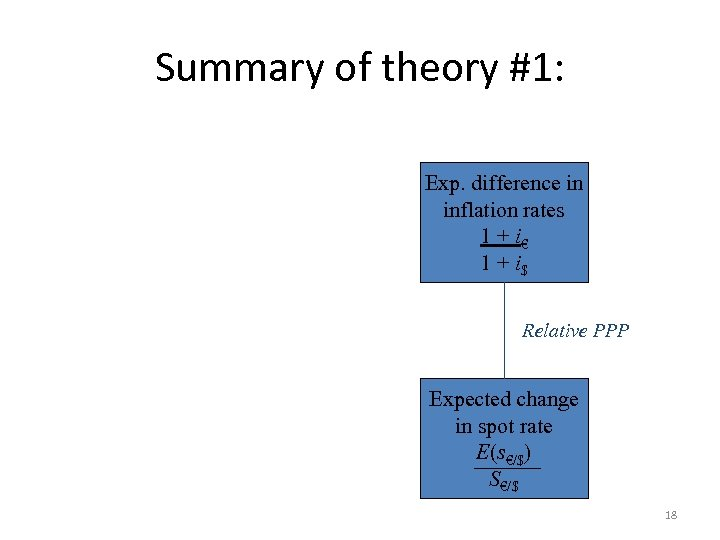Summary of theory #1: . Exp. difference in inflation rates 1 + i€ 1 + i\$ Relative PPP Expected change in spot rate E(s€/\$) S€/\$ 18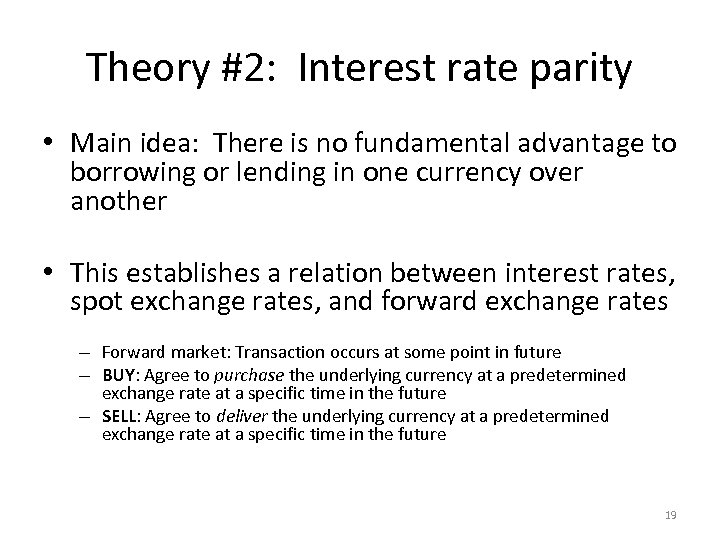Theory #2: Interest rate parity • Main idea: There is no fundamental advantage to borrowing or lending in one currency over another • This establishes a relation between interest rates, spot exchange rates, and forward exchange rates – Forward market: Transaction occurs at some point in future – BUY: Agree to purchase the underlying currency at a predetermined exchange rate at a specific time in the future – SELL: Agree to deliver the underlying currency at a predetermined exchange rate at a specific time in the future 19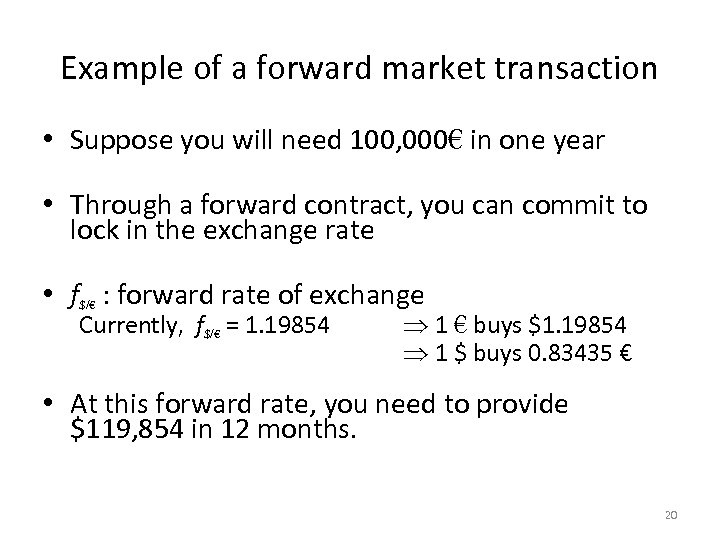Example of a forward market transaction • Suppose you will need 100, 000€ in one year • Through a forward contract, you can commit to lock in the exchange rate • f\$/€ : forward rate of exchange Currently, f\$/€ = 1. 19854 1 € buys \$1. 19854 1 \$ buys 0. 83435 € • At this forward rate, you need to provide \$119, 854 in 12 months. 20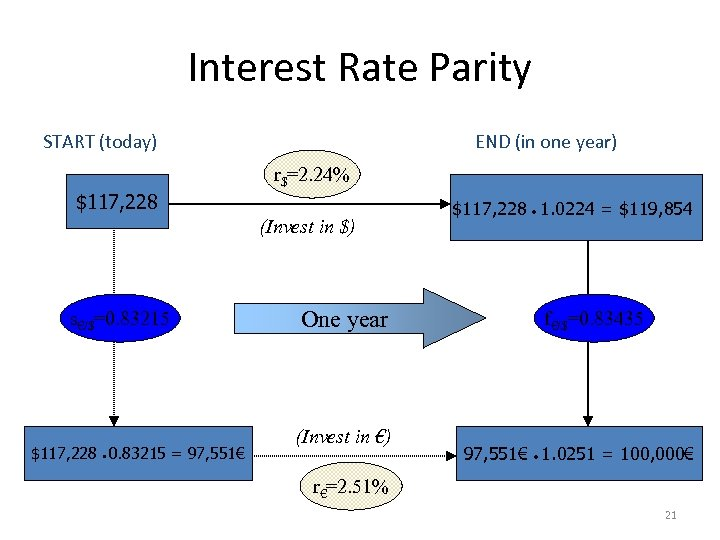Interest Rate Parity START (today) \$117, 228 END (in one year) r\$=2. 24% (Invest in \$) s€/\$=0. 83215 \$117, 228 0. 83215 = 97, 551€ One year (Invest in €) \$117, 228 1. 0224 = \$119, 854 f€/\$=0. 83435 97, 551€ 1. 0251 = 100, 000€ r€=2. 51% 21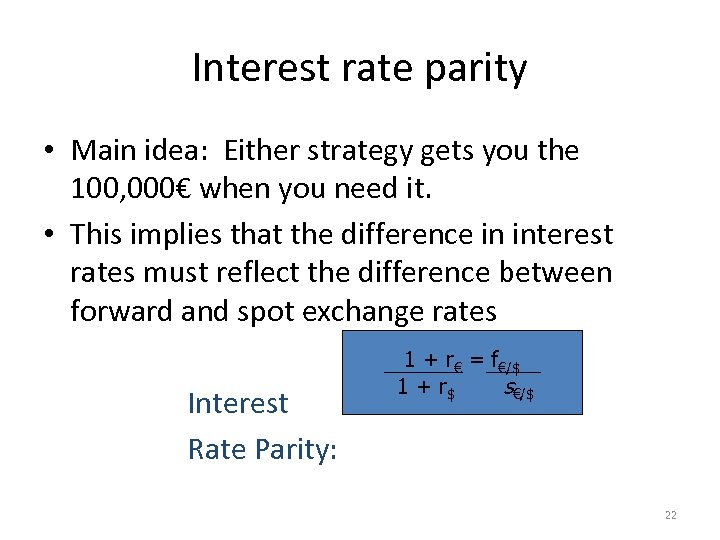Interest rate parity • Main idea: Either strategy gets you the 100, 000€ when you need it. • This implies that the difference in interest rates must reflect the difference between forward and spot exchange rates Interest Rate Parity: 1 + r€ = f€/\$ 1 + r\$ s€/\$ 22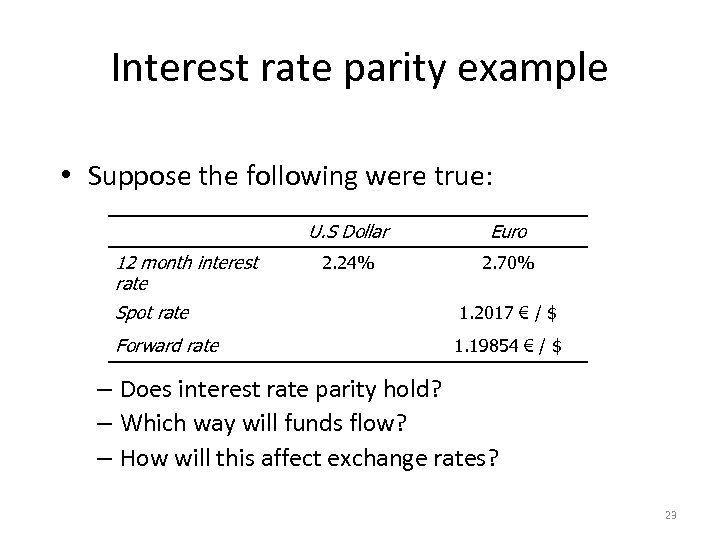Interest rate parity example • Suppose the following were true: U. S Dollar 12 month interest rate Euro 2. 24% 2. 70% Spot rate 1. 2017 € / \$ Forward rate 1. 19854 € / \$ – Does interest rate parity hold? – Which way will funds flow? – How will this affect exchange rates? 23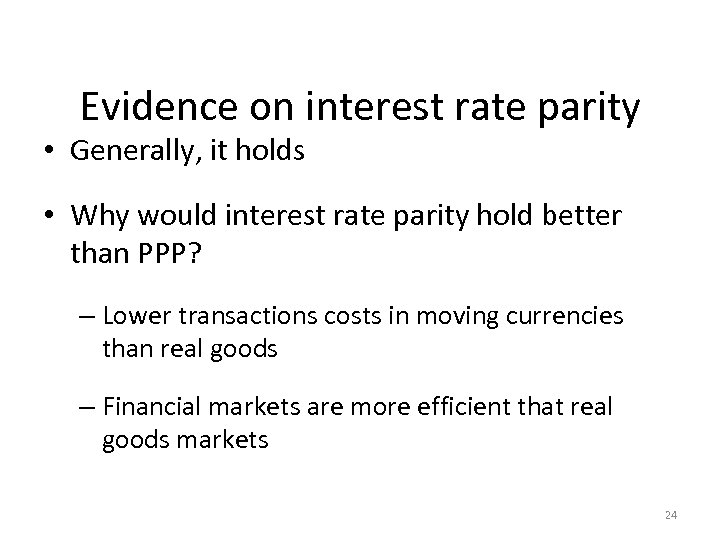Evidence on interest rate parity • Generally, it holds • Why would interest rate parity hold better than PPP? – Lower transactions costs in moving currencies than real goods – Financial markets are more efficient that real goods markets 24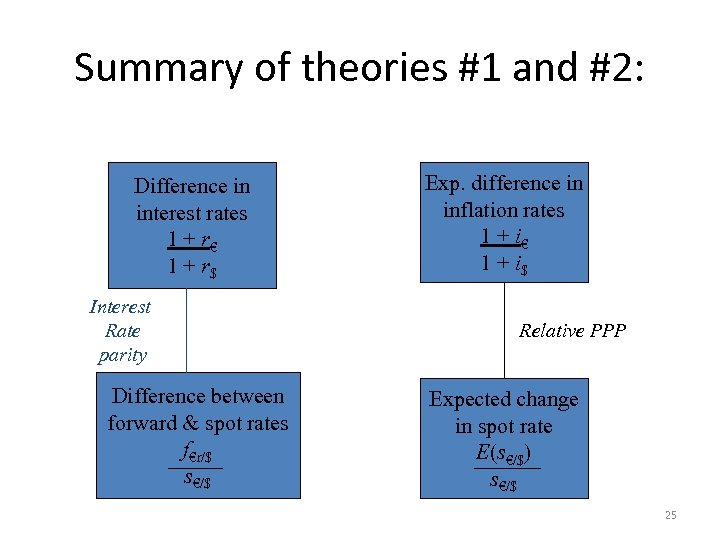Summary of theories #1 and #2: . Difference in interest rates 1 + r€ 1 + r\$ Interest Rate parity Difference between forward & spot rates f€r/\$ s€/\$ Exp. difference in inflation rates 1 + i€ 1 + i\$ Relative PPP Expected change in spot rate E(s€/\$) s€/\$ 25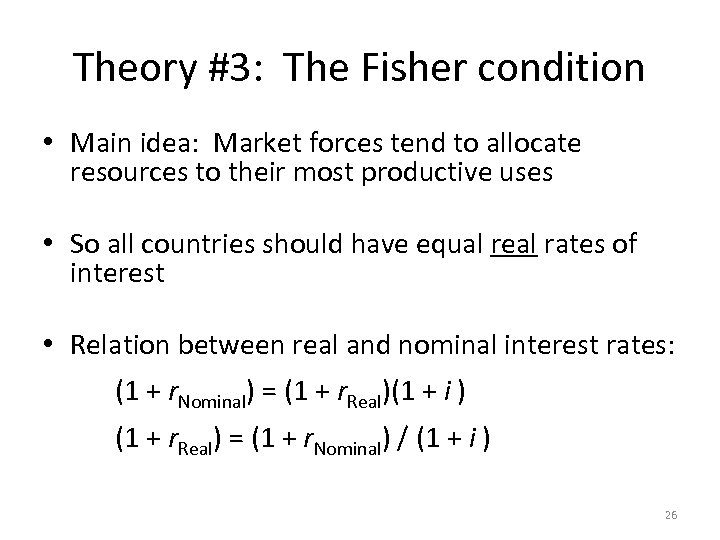Theory #3: The Fisher condition • Main idea: Market forces tend to allocate resources to their most productive uses • So all countries should have equal real rates of interest • Relation between real and nominal interest rates: (1 + r. Nominal) = (1 + r. Real)(1 + i ) (1 + r. Real) = (1 + r. Nominal) / (1 + i ) 26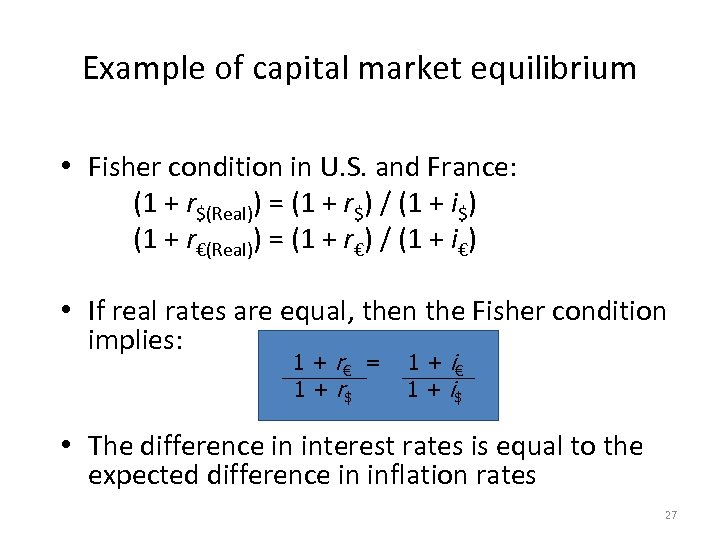Example of capital market equilibrium • Fisher condition in U. S. and France: (1 + r\$(Real)) = (1 + r\$) / (1 + i\$) (1 + r€(Real)) = (1 + r€) / (1 + i€) • If real rates are equal, then the Fisher condition implies: 1 + r€ = 1 + r\$ 1 + i€ 1 + i\$ • The difference in interest rates is equal to the expected difference in inflation rates 27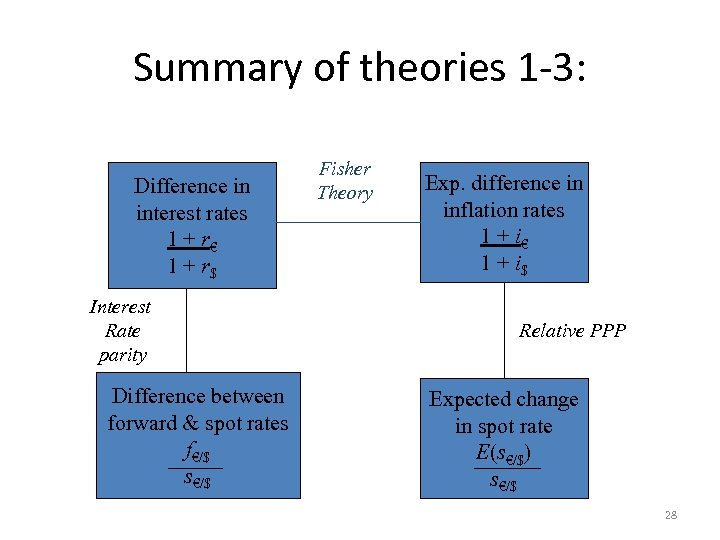Summary of theories 1 -3: . Difference in interest rates 1 + r€ 1 + r\$ Interest Rate parity Difference between forward & spot rates f€/\$ s€/\$ Fisher Theory Exp. difference in inflation rates 1 + i€ 1 + i\$ Relative PPP Expected change in spot rate E(s€/\$) s€/\$ 28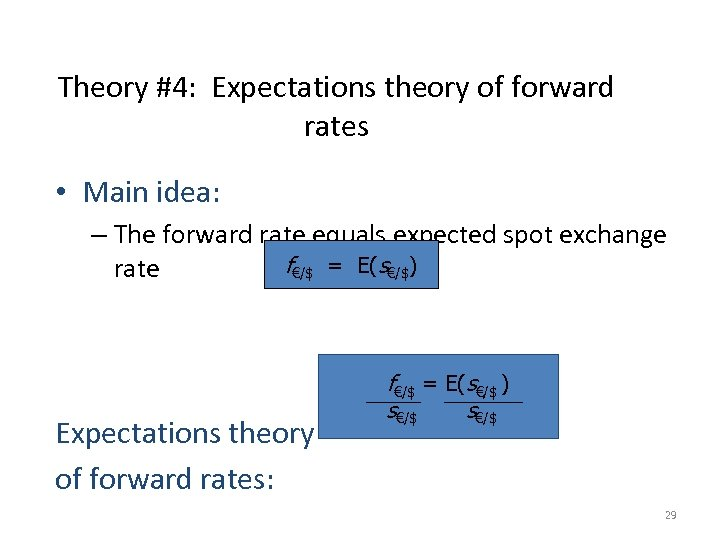Theory #4: Expectations theory of forward rates • Main idea: – The forward rate equals expected spot exchange f€/\$ = E(s€/\$) rate Expectations theory of forward rates: f€/\$ = E(s€/\$ ) s€/\$ 29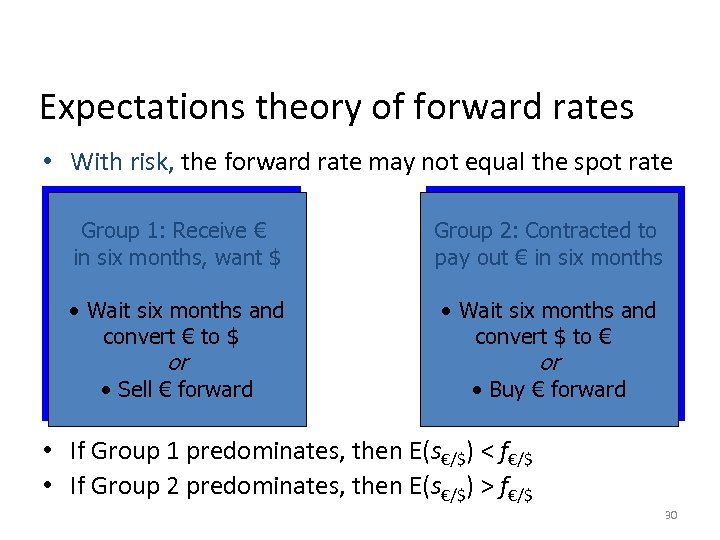Expectations theory of forward rates • With risk, the forward rate may not equal the spot rate Group 1: Receive € in six months, want \$ Group 2: Contracted to pay out € in six months • Wait six months and convert € to \$ or • Sell € forward • Wait six months and convert \$ to € or • Buy € forward • If Group 1 predominates, then E(s€/\$) < f€/\$ • If Group 2 predominates, then E(s€/\$) > f€/\$ 30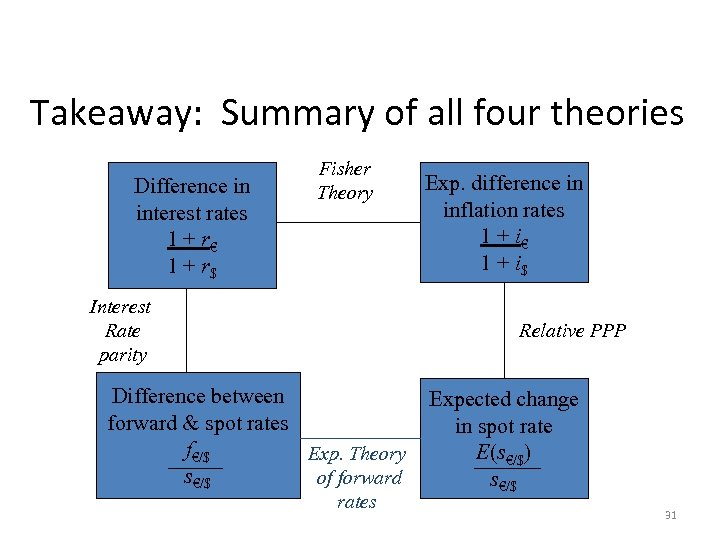Takeaway: Summary of all four theories. Difference in interest rates 1 + r€ 1 + r\$ Fisher Theory Interest Rate parity Exp. difference in inflation rates 1 + i€ 1 + i\$ Relative PPP Difference between forward & spot rates f€/\$ Exp. Theory s€/\$ of forward rates Expected change in spot rate E(s€/\$) s€/\$ 31